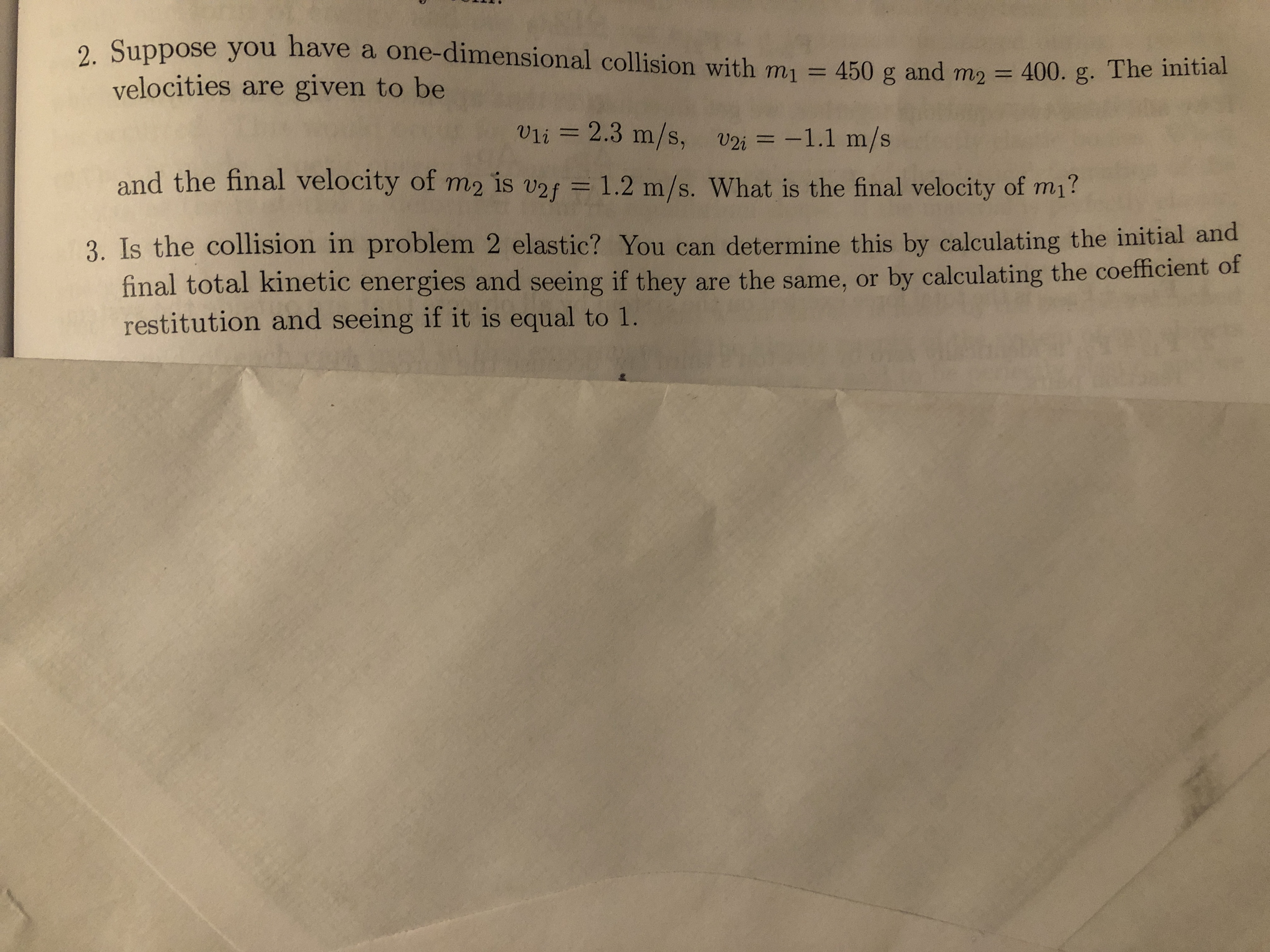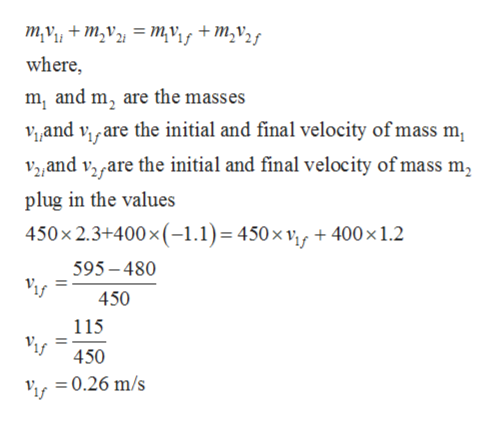# 2. Suppose you have a one-dimensional collision with m1 = 450 g and m2 400. g. The initialvelocities are given to beVli 2.3 m/s, v2i -1.1 m/sand the final velocity of m2 is v2f = 1.2 m/s. What is the final velocity of mi?3. Is the collision in problem 2 elastic? You can determine this by calculating the initial andfinal total kinetic energies and seeing if they are the same, or by calculating the coefficient ofrestitution and seeing if it is equal to 1.

Questionhelp_outlineImage Transcriptionclose2. Suppose you have a one-dimensional collision with m1 = 450 g and m2 400. g. The initial velocities are given to be Vli 2.3 m/s, v2i -1.1 m/s and the final velocity of m2 is v2f = 1.2 m/s. What is the final velocity of mi? 3. Is the collision in problem 2 elastic? You can determine this by calculating the initial and final total kinetic energies and seeing if they are the same, or by calculating the coefficient of restitution and seeing if it is equal to 1. fullscreen
check_circleExpert Solution
Step 1

Given:

For m1:

Mass = 450 g

Initial velocity = 2.3 m/s

Final velocity = ?

For m2:

Mass = 400 g

Initial velocity = -1.1 m/s

Final velocity = 1.2 m/s

Step 2

According to the law of conservation of momentum.

Total momentum bef...help_outlineImage Transcriptionclosem,v+m2v = mvif +M,v2g where m, and m, are the masses Vand viare the initial and final velocity of mass m V2and vyare the initial and final velocity of mass m, plug in the values 450x 2.3+400x (-1.1)= 450 x vf +400x 1.2 595-480 450 115 450 =0.26 m/s fullscreen

### Want to see the full answer?

See Solution

#### Want to see this answer and more?

Solutions are written by subject experts who are available 24/7. Questions are typically answered within 1 hour*

See Solution
*Response times may vary by subject and question
Tagged in

### Work,Power and Energy